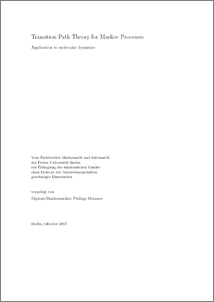Repository: Freie Universität Berlin, Math Department

# Transition Path Theory for Markov Processes

Metzner, Ph. (2007) Transition Path Theory for Markov Processes. PhD thesis, Free University of Berlin.Preview
PDF - Published Version
Available under License Creative Commons Attribution Non-commercial.

31MB

## Abstract

In this thesis, we present the framework of transition path theory (TPT) for time continuous Markov processes with continuous and discrete state space. TPT provides statistical properties of the ensemble of reactive trajectories between some start and target sets and yields properties such as the committor function, the probability distribution of the reactive trajectories, their probability current and their rate of occurrence. We shown that knowing these objects allows one to arrive at a complete understanding of the mechanism of the reaction. The main objects of TPT for Markov diffusion processes are explicitly derived for the Langevin and Smoluchowski dynamics and illustrate them on a various number of low-dimensional examples. Despite the simplicity of these examples compared to those encountered in real applications, they already demonstrate the ability of TPT to handle complex dynamical scenarios. The main challenge in TPT for diffusion processes is the numerical computation of the committor function as a solution of a Dirichlet-Neumann boundary value problem involving the generator of the process. Beside the derivation of TPT for Markov jump processes, we focus on the development of efficient graph algorithms to determine reaction pathways in discrete state space. One approach via shortest-path algorithms turns out to give only a rough picture of possible reaction channels whereas the network approach allows a hierarchical decomposition of the set of reaction pathways such that the dominant channels can be identified. We successfully apply the latter approach to an example of conformational dynamics of a bio-molecule. In particular, we make use of a maximum likelihood method to estimate the infinitesimal generator of a jump process from an incomplete observation. Finally, we address the question of error propagation in the committor function computation for Markov chains.

Item Type: Thesis (PhD) Mathematical and Computer Sciences > Mathematics Department of Mathematics and Computer Science > Institute of MathematicsDepartment of Mathematics and Computer Science > Institute of Mathematics > BioComputing Group 159 BioComp Admin 29 Jan 2009 16:43 03 Mar 2017 14:40

Repository Staff Only: item control page